# Problem Solver II

Grade Levels: 1-6
This series of six books guides students through step-by-step instruction in mathematical problem solving. Ten valuable problem-solving strategies are introduced and students learn how to apply each strategy using a 4-step method.## About the Program

Problem Solver II builds on the concepts introduced in The Problem Solver. Problem Solver II offers hundreds of new problems that can be integrated into any math curriculum, and uses the same ten strategies and four-step method of problem solving. All problems are keyed to math strands and skills, thus allowing teachers to customize the program to meet the needs of students.

Features:

• Easy-to use format demonstrates how to guide students through the problem-solving process
• Introduces and explains 10 widely accepted problem-solving strategies that can be applied in a variety of problem-solving situations
• Presents different types of problems, identifies strategies to use to solve them, and models thinking involved in using the strategies
• Helps students develop a systematic approach to problem solving through consistent use of a 4-step method
• Identifies mathematical content areas and specific mathematics skills addressed by each problem
• Prepares students for answering extended-response test items by having them explain their thinking
• Provides a bank of problems for additional practice in applying strategies using the 4-step method
• Includes information on assessing progress

## Program Components

•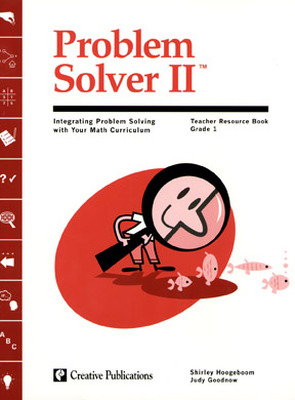Problem Solver II: Grade 1 Teacher Guide
•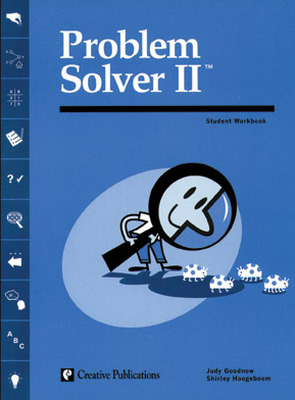Problem Solver II: Grade 2 Student Book (Set of 5)
•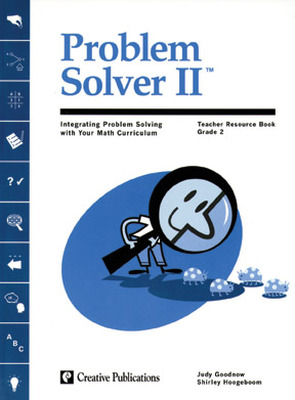Problem Solver II: Grade 2 Teacher Guide
•Problem Solver II: Grade 4 Classroom Set
•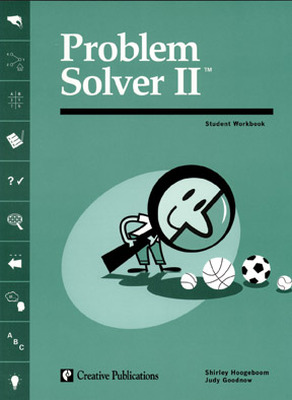Problem Solver II: Grade 4 Student Book (Set of 5)
•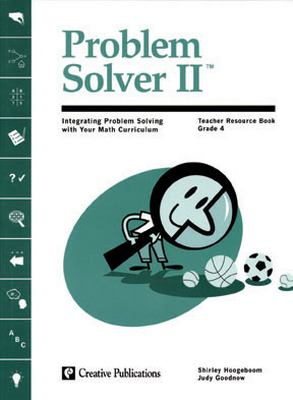Problem Solver II: Grade 4 Teacher Guide
•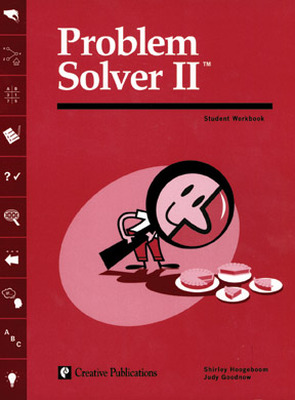Problem Solver II: Grade 5 Student Book (Set of 5)
•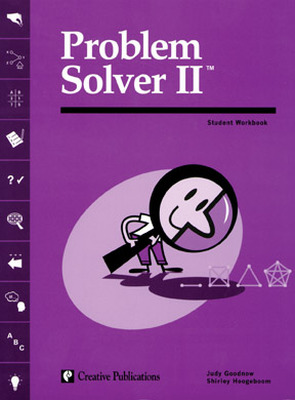Problem Solver II: Grade 6 Student Book (Set of 5)
•Problem Solver 2, Grade 2 Workbook
•Problem Solver 2, Grade 3 Workbook
•Problem Solver 2, Grade 4 Workbook
•Problem Solver 2, Grade 5 Workbook
•Problem Solver 2, Grade 6 Workbook
Sort by:

## Products

To learn about the specific purchase options for this program, please contact our Customer Service team at 800-338-3987

Sample Lessons

Student Edition

Teacher Edition